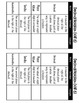# 4th Grade Fraction Vocabulary ChartsSubject
Resource Type
File Type

PDF

(2 MB)
Product Rating
Standards
• Product Description
• StandardsNEW

Need a way to help your students learn and have access to new math vocabulary? These charts are the perfect solution for that! These charts are a great way to introduce new math vocabulary words and have as a reference for your students. These charts work well in a math notebook or as a math center. They come with 3 versions: pre-filled definition with examples, pre-filled definition with blank examples and fill-in-the-blank definitions.

Bundle Includes (41 Charts in Total):

-4.NF.1 Equivalent Fractions (Filled, Partially Filled and Fill-In-the Blank)

-4.NF.2 Comparing Fractions (Filled, Partially Filled and Fill-In-the Blank)

-4.NF.3 Adding and Subtracting Fractions (Filled, Partially Filled and Fill-In-the Blank)

-4.NF.4 Multiplying Fractions (Filled, Partially Filled and Fill-In-the Blank)

-4.NF.5 Denominators of 10 and 100 (Filled, Partially Filled and Fill-In-the Blank)

-4.NF.6 Decimal Notation (Filled, Partially Filled and Fill-In-the Blank)

-4.NF.7 Comparing Decimals (Filled, Partially Filled and Fill-In-the Blank)

Thank you for checking out this resource! Please leave feedback if you choose to purchase this resource!

Compare two decimals to hundredths by reasoning about their size. Recognize that comparisons are valid only when the two decimals refer to the same whole. Record the results of comparisons with the symbols >, =, or <, and justify the conclusions, e.g., by using a visual model.
Use decimal notation for fractions with denominators 10 or 100. For example, rewrite 0.62 as 62/100; describe a length as 0.62 meters; locate 0.62 on a number line diagram.
Express a fraction with denominator 10 as an equivalent fraction with denominator 100, and use this technique to add two fractions with respective denominators 10 and 100. For example, express 3/10 as 30/100, and add 3/10 + 4/100 = 34/100.
Solve word problems involving multiplication of a fraction by a whole number, e.g., by using visual fraction models and equations to represent the problem. For example, if each person at a party will eat 3/8 of a pound of roast beef, and there will be 5 people at the party, how many pounds of roast beef will be needed? Between what two whole numbers does your answer lie?
Understand a multiple of 𝘢/𝘣 as a multiple of 1/𝘣, and use this understanding to multiply a fraction by a whole number. For example, use a visual fraction model to express 3 × (2/5) as 6 × (1/5), recognizing this product as 6/5. (In general, 𝘯 × (𝘢/𝘣) = (𝘯 × 𝘢)/𝘣.)
Total Pages
N/A
N/A
Teaching Duration
N/A
Report this Resource to TpT
Reported resources will be reviewed by our team. Report this resource to let us know if this resource violates TpT’s content guidelines.